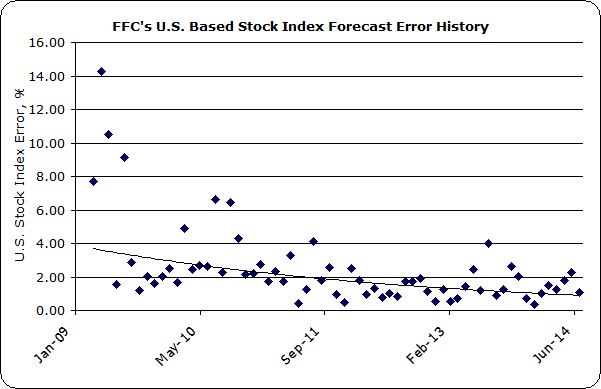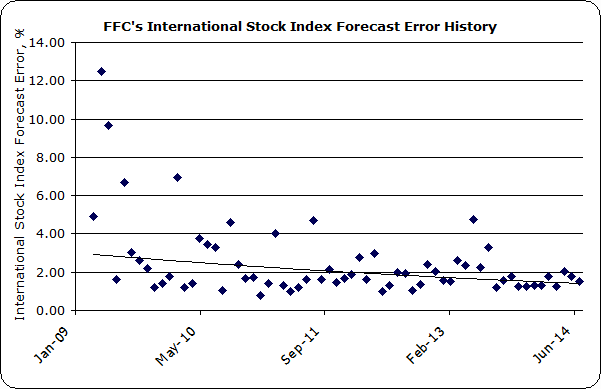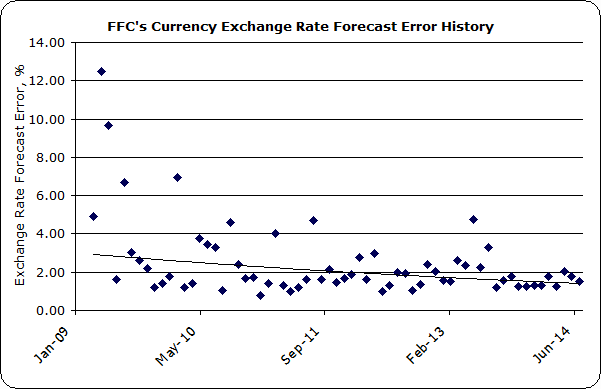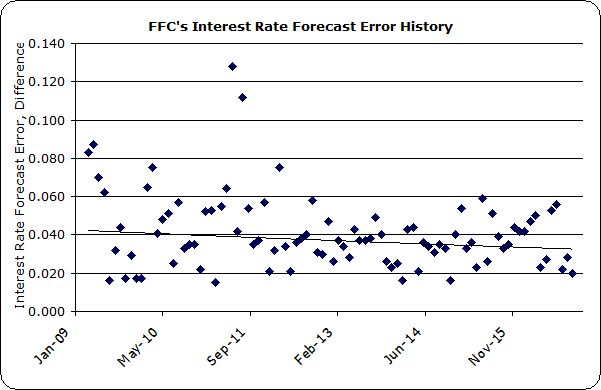﻿ FFC's Forecast Accuracy

The

Since 1997

# Financial Forecast Center's Forecast Accuracy

## U.S. Stock Index Forecast Accuracy

Below is a chart of the Financial Forecast Center's forecast accuracy of all U.S. based stock indexes, including those of Dow Jones, Standard and Poor's, Russell, and Wilshire.  The chart is the percent difference between the forecast values and actual values for the designated period across all U.S. stock index forecasts published by FFC.From the graph of FFC's stock index forecast errors, you can see an overall improvement in the accuracy of the forecasts since the Great Recession of 2008-2009.  The downward sloping line in the graph above is the trendline.  For reference, the average over the last five year period is 1.97%.

## International Stock Index Forecast Accuracy

Below is a chart of the Financial Forecast Center's forecast accuracy of all International stock indexes, including the Nikkei, Hang Seng, DAX, CAC, FTSE, IPC and Straits Times. Again, the chart is the percent difference between the forecast values and actual values for the designated period across all international stock index forecasts published by FFC.From the graph of FFC's stock index forecast errors, again you can see an overall improvement in the accuracy of the forecasts since the global financial market turmoil of 2008-2009.  The downward sloping line in the graph above is the trendline.  For reference, the average over the last five year period is 2.09%.

## Currency Exchange Rate Forecast Accuracy

Below is a chart of the Financial Forecast Center's forecast accuracy of all currency exchange rates, including the yen, euro, yuan, pound and aussie. Again, the chart is the percent difference between the forecast values and actual values for the designated period across all currency forecasts published by FFC.From the graph of FFC's FX forecast errors, you can see a slight overall improvement in the accuracy of the forecasts over the period from 2009 to present.  The downward sloping line in the graph above is the trendline.  For reference, the average over the last five year period is 0.78%.

## Interest Rate Forecast Accuracy

Below is a chart of the Financial Forecast Center's accuracy of all interest rate forecasts, including U.S. treasury securities and LIBORs. The chart is the difference between the actual values and forecast values for the designated period across all interest rate forecasts published by FFC.From the graph of FFC's interest rate forecast errors, you can see a slight overall improvement in the accuracy of the forecasts over the period from 2009 to present.  The downward sloping line in the graph above is the trendline.  For reference, the average over the last five year period is 0.038 (3.8 basis points).

And for those asking themselves "yeah, but how well does this work past one month?", here is a prior long range forecast of the S&P 500 over a 36 month window.

Popular Forecasts